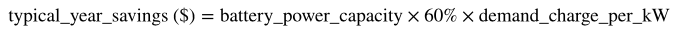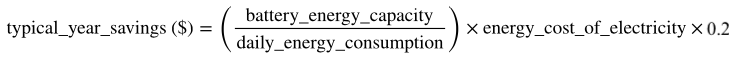Station A Knowledge Base

# How does Station A calculate the financials for a battery storage system?

## We calculate the financials of a battery storage system by calculating the battery system size, peak demand reduction, and annual cost.

The financials of a battery storage system are represented as typical savings, or the percentage utility bill reduction we expect, net of the annual amortized cost of the battery.

To get to this value, we calculate the battery system size, as described above, along with the following two other crucial intermediate numbers (with more detail behind these calculations below):

1. Peak demand reduction: The amount that the battery could reduce peak demand during a typical time of use period
2. Annual cost: The cost of the battery storage system, amortized to an annual basis

### Peak Demand Reduction

We model peak demand reduction based on a simple 60% performance. In other words, if a building installs a 100 kW battery behind the meter, we assume that, on average, their peak demand is reduced by 60 kW in each time-of-use period.

We next assume that the peak load reduction linearly reduces the demand charge costs and does not affect the energy and fixed charges on the customer's bill. This allows us to calculate typical year savings:### Energy Arbitrage Savings

Additionally, we assume that the battery can save cost by moving customer energy consumption out of high-cost time of use periods into low-cost periods. We calculate these savings by calculating the percentage of all energy use that the battery can store (and thus redistribute), the energy cost of electricity on the building's tariff, and an assumption that energy consumption can be moved to a time of user period that is 20% cheaper.### Typical Bill Savings

We can then calculate bill savings for a typical year by simply subtracting the costs listed above from the savings listed above.

### Typical Energy Savings

The only impact we model from battery energy storage is round-trip efficiency losses, so energy savings are always negative.